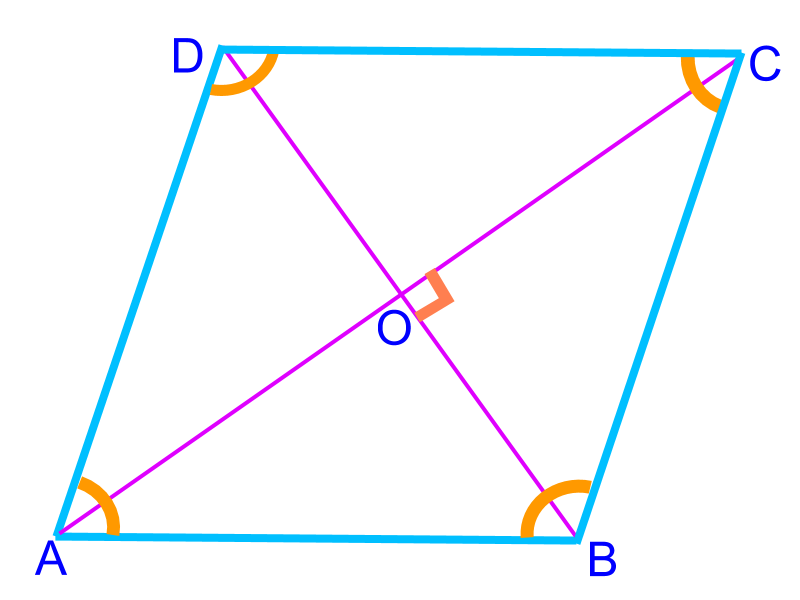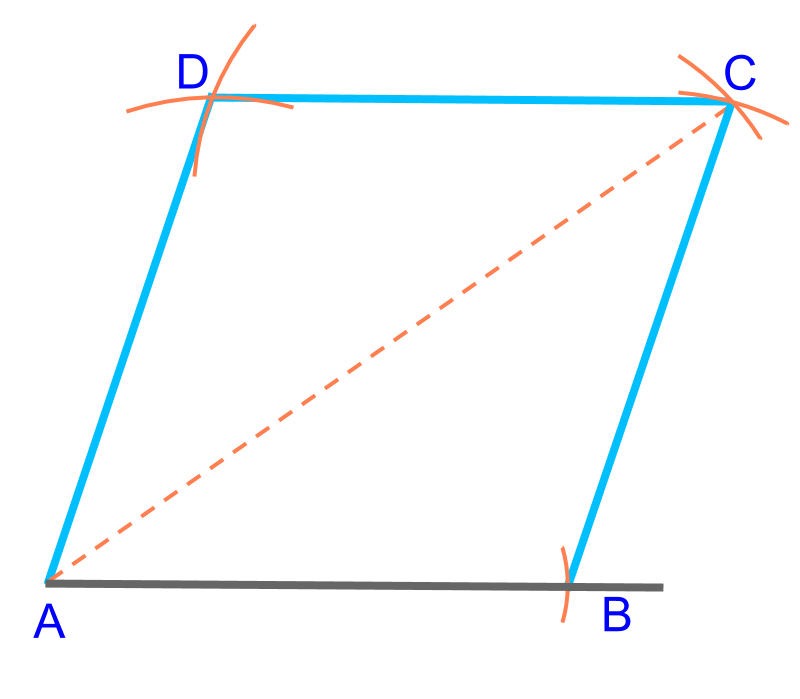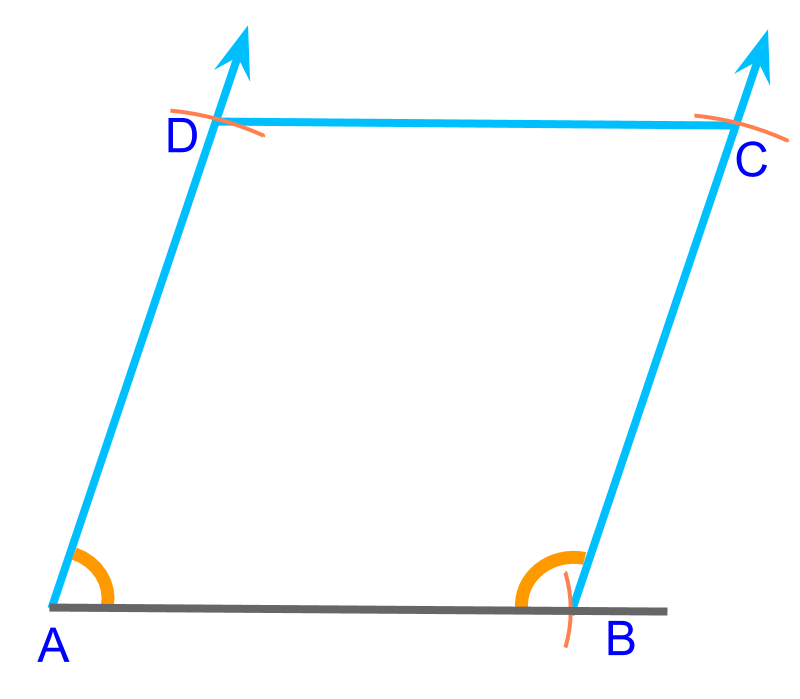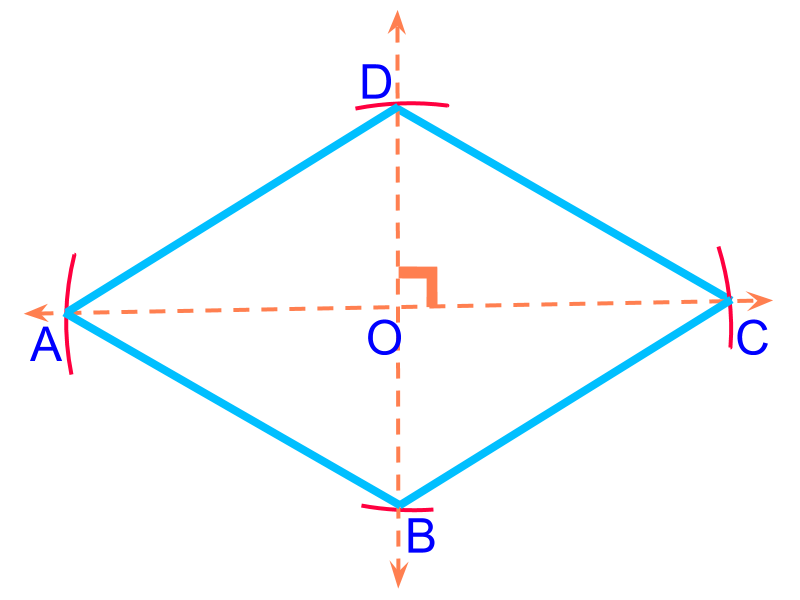maths > construction-basics

Construction of Rhombus

what you'll learn...

overview

In this page, constructing rhombus is explained. It is outlined as follows.

•  Properties of rhombus is explained

•  The number of independent parameters in a rhombus is 2$2$

•  For a given parameter, construction of rhombus is approached as combination of triangles (sss, sas, asa, rhs, sal) and using the properties of rhombus.

understanding rhombus

A rhombus is a parallelogram with all sides equal.A quadrilateral is defined by 5$5$ parameters. A parallelogram is defined by 3$3$ parameters. And in a rhombus, the following properties provide additional dependency of parameters

•  All sides are equal (and parallel)

•  the diagonals perpendicularly bisect

•  opposite angles are equal

These properties cause one parameter dependent on other parameters and effectively, a rhombus is defined by 2$2$ parameters.To construct a rhombus, a side (¯¯¯¯¯¯AB$\overline{A B}$) and a diagonal (¯¯¯¯¯¯AC$\overline{A C}$) are given. This is illustrated in the figure. To construct, consider this as two SSS triangles $A B C$ and $A C D$To construct a rhombus, a side ($\overline{A B}$) and an angle ($\angle B$) are given. This is illustrated in the figure.

To construct the specified rhombus, "Consider this as two SAS triangles $A B C$ and $B A D$". Use the property $\angle A + \angle B = {180}^{\circ}$ to find the second angle.To construct a rhombus, two diagonals ($\overline{A C}$, $\overline{B D}$) are given. This is illustrated in the figure.

To construct the specified rhombus, "Consider this as two SAS triangles $D O C$ and $A O B$". Use the property that the diagonals perpendicularly bisect and mark the vertices at half diagonals.

summaryConstruction of Rhombus :

Properties of Rhombus

•  All sides are equal (and parallel)

•  the diagonals perpendicularly bisect

•  opposite angles are equal

•  $1$ side and $1$ diagonal
•  $1$ side and $1$ angle
•  $2$ diagonals